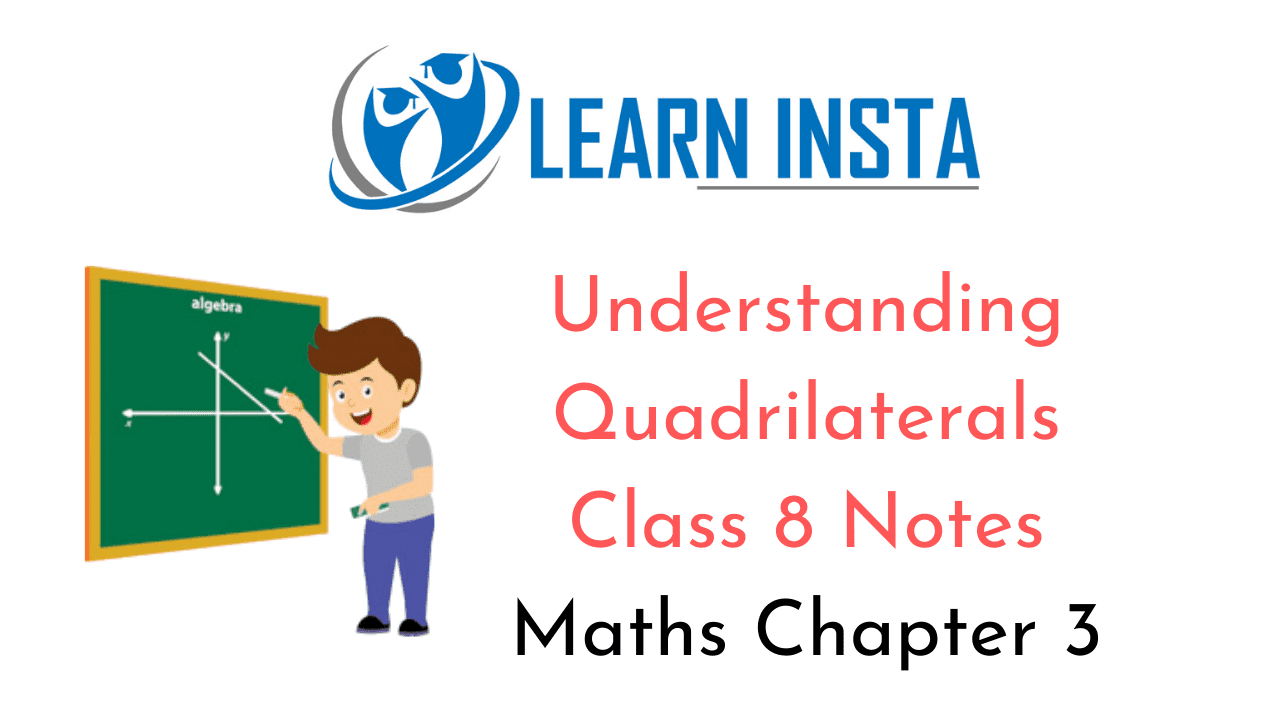On this page, you will find Understanding Quadrilaterals Class 8 Notes Maths Chapter 3 Pdf free download. CBSE NCERT Class 8 Maths Notes Chapter 3 Understanding Quadrilaterals will seemingly help them to revise the important concepts in less time.

## CBSE Class 8 Maths Chapter 3 Notes Understanding Quadrilaterals

### Understanding Quadrilaterals Class 8 Notes Conceptual Facts

Polygon: A simple closed curve made up of only line segments is called a polygon.
Examples of Polygons:
(i) Triangle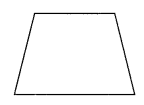(iii) Pentagon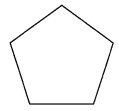(iv) HexagonConvex and concave polygonsRegular and irregular polygons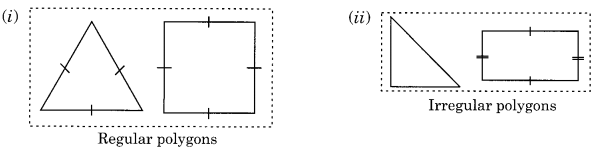Angle sum property: The sum of three angles of a triangle is 180° In AABC, ∠A + ∠B + ∠C = 180°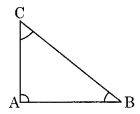Sum of all the exterior angles of a polygon is 360°. In the given polygon ABODE, exterior angles ∠1 + ∠2 + ∠3 + ∠4 + ∠5 = 360°.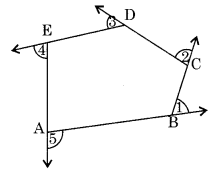(i) ParallelogramProperties
(a) Opposite angles are equal
(b) Opposite sides are equal
(c) Diagonals bisect each other

(ii) Rhombus:(a) All sides are equal
(b) Opposite angles are equal
(c) Diagonals bisect each other at 90°

(iii) Rectangle(a) It is a parallelogram having each angle of 90°
(b) Opposite sides are equal
(c) Diagonals are equal

(iv) Square: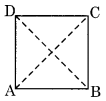(a) All sides are equal
(b) Each angle is of 90°
(c) Diagonals are equal and bisect each other at 90°

(v) Kite: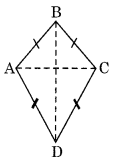(a) Diagonals are perpendicular to each other.
(b) One of the diagonals bisects the other
(c) m∠A = m∠C but m∠B ≠ m∠D

(vi) Trapezium: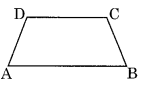A pair of opposite sides is parallel to each other.